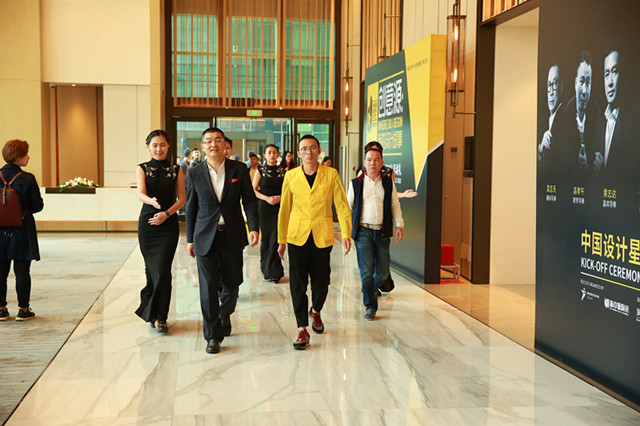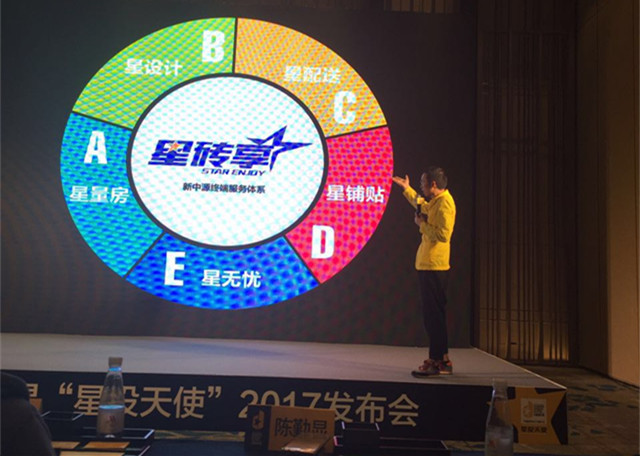|

# 发起H+,又做星投天使,新中源不“卖砖”了?

2017年3月15日，深圳，中州万豪酒店，灯光闪烁，2017-2018中国设计星启动礼在这里启幕。

活动现场，一群年轻的设计师在入口处拍合照，年轻帅气的男孩子们大声喊：帅不帅？旁边走过的华夏陶瓷网总编辑刘小明回应：“帅！”这是一场为他们举办的活动，更像是一个年轻人的秀场，会场外面两面巨大的背景墙上，一面是各位名满业界的大牌设计师组成的明星导师团队，一面是设计星往届获奖选手展示，这是一场由新中源陶瓷和广州设计周发起的造星运动，尤其是当明星导师步入场地的时候，全场欢呼，掌声雷动，让人想起中国好声音。这样的场面对新中源团队来说已经非常熟悉了，因为他们已经连续第三年举办这样的活动，每一年的投入都在千万以上，费用之高，规模之大，让业界咂舌。华夏陶瓷网总编辑刘小明说：“现在的设计师活动不好做，新中源这个是人气最旺的。”活动现场的星光大道，是用新中源BIG+新品白色大板铺成的，这让众创社创始人罗杰想起了前阵子另外一个企业推广的大板：更大更高端。

去中心化、平台化思维的中国设计星

但是在新中源团队的眼里，中国设计星并不是单纯的设计师活动，更不是产品推广，新中源陶瓷常务副总陈勤显这样解释：“中国设计星是新锐设计平台，用的是去中心化，平台化思维，大家在这个平台上都要受益，不管是顾问导师，还是执行导师，还是选手，甚至消费者”，在陈勤显看来，通过参与中国设计星，组织中国设计星，新中源更能读懂消费者，同时要求经销商能读懂设计，能够在终端提供更好的设计方案给消费者，同时对于真正牛逼的消费者，依托中国设计星的选手提供牛逼的设计服务，“未来三到五年，新中源会通过共享和分享的概念，扶持一些有能力的大客户，给消费者提供一套标准化服务，从而保证中国设计星的设计营销服务能力能够落地”。在发布会现场，陈勤显特意换上了黄色休闲西装，黑色哈伦裤，运动鞋，广州设计周总干事张宏毅问台下的观众：“大家有没有注意到他今天穿的这身衣服？什么颜色？”观众：“黄色！”张宏毅：“错！中国设计星色！”一旁的陈勤显回应：“中国设计星的主色调是是黄和黑，所以我今天穿来，我们要跟大家交一辈子的朋友，谢谢大家！”“交一辈子的朋友”这并不是一句空话，因为新中源已经有了一套构想并且已经按照计划在有步骤的执行这个构想。

星投天使是个天使基金吗？

这次发布会的主题是中国设计星“星投天使”，陈勤显是这么解释“星投天使”的：“新中源公司计划拿500到1000万元，支持有能力的设计师，可以做产品美学顾问，也可以做空间美学顾问，也可以成为第三方美学顾问，只要有能力，一定能得到这份回报，因为新中源就是说实话干实事，只要你有能力我们就支持你。”在现场，广州设计周总干事张宏毅和陈勤显都提到了去年就启动的“H+”，对于星投天使和H+的关系，陈勤显这么解释：“H+是星投天使推动的一个项目落地的表现，H+有很多种，但助推H+落地的只有星投天使，H+是一个实物要落地，让千家万户看得到，而星投天使是助推它飞起的一股风，我们今年投了一百万开发一个软件，希望能够使中国设计星像优步软件一样，带来的不仅是思想上、利益上的成长，更多的是落地项目的成长，以后所有的设计师都可以利用这个平台去推广，去合作。”陈勤显提到的这款软件已经开始接受设计师报名，未来所有的设计师都可以在这个平台上找业主，找材料，业主也可以通过这个平台找到设计师，找到材料，材料供应商也可以随时为业主和设计师提供支持和帮助。

广州设计周总干事张宏毅从另外一个角度解释了星投天使和H+。张宏毅表示，H+就是从家居到酒店范围来做概念，有关产品美学、空间美学、大众用户美学三方面的入口，也就是未来H+这个入口，是产品、空间、消费者所需要选择的一个价值入口，作为用户，可以采购到自己需要的东西，作为设计师，可以发挥自己的价值，作为品牌商，可以在这里找到着陆点，这个平台很开放，谁都可以来发现设计的价值，“我们的目标是驱动产业升级，创造人类幸福。”张宏毅的接任者、广州设计周下一任总干事贺文广则给出了更具体的答案：“2017中国设计星首个星投天使——新中源陶瓷，将拿出10家托斯卡纳品牌形象店作为首批星投天使项目面向2017中国设计星参展设计师招募设计合作，”贺文广表示，所有有项目想找有创意活力的年轻设计师们做设计，但是又找不到合适设计师的业主们，欢迎申报成为2017中国设计星星投天使，不论你是精品酒店、民宿、餐饮店、商店、展厅、还是住宅等室内项目，中国设计星总有那么多优质“年轻款”适合你。

“新中源要跟设计师交一辈子的朋友”

作为中国设计星的主办方，新中源陶瓷要做的显然不只是卖砖这么简单。陈勤显明确表示：“新中源今天是卖砖，未来不一定只是卖砖”，从2017年开始，新中源要做一个星砖享体系，包括星量房、星无忧、星铺贴、星配送、星设计，“我们要在全过程当中给一套SOP（标准操作程序），保证新中源陶瓷全程无忧，2017年新中源要走向前端，构建一个以消费者满意为中心的一个载体，叫星砖享终端服务体系，新中源要做的不只是一场设计师活动，要做的是跟中国设计师永远走在一起。”在发布会现场，华夏陶瓷网总编辑刘小明问了陈勤显这样一个问题：“是不是其他材料商也可以加入进来？”陈勤显表示：“只要是对新中源的产品品类有补充，都可以考虑，当然也要首先考虑新中源本身的盈利。”这个问题的探讨延续到了台下，在午餐休息的间隙，众创社创始人罗杰问新中源市场总监郭浩：“如果你们不是做陶瓷的，这个平台是不是可以做更多事情，可以做的更好？”

事实上，的确有风投对新中源的H+项目表现出了浓厚的兴趣，但是被陈勤显拒绝了。在外界看来，这是一个陶瓷企业做出了比互联网公司更互联网思维的项目，在陈勤显看来，“互联网”实际上不存在你是在做互联网还是实体经济，“就像今天我们的渠道模式也要走互联网思维，要共享分享，今年我们在推动我们的代理商走共享分享模式，也是一样的，我们不要固化于互联网就是解决互联网的问题，互联网是一种思维，是一种流动价格和各方面流动的思维，一定要这样理解”。

“当准备就绪，一切为时已晚”

对新中源而言，无论是推H+，星投天使，或者是推出托斯卡纳、推出BIG+系列，都更像是对未来的一种投资和布局，陈勤显表示，2017、2018、2019这三年对新中源来说很关键，“新中源如果能踏上一个台阶，预示着新中源一定会成为这个行业的一条龙”。

新中源南昌未来店设计师邵唯晏说了这样一句话：“当准备就绪，一切为时已晚。”这句话值得玩味。处在一个瞬息万变的时代，如何未雨绸缪成为每个企业都面临的考验。从这个角度出发，“未来不一定只是卖砖”的新中源也许是最终赢家。

`声明：本文由入驻焦点开放平台的作者撰写，除焦点官方账号外，观点仅代表作者本人，不代表焦点立场错误信息举报电话： 400-099-0099，邮箱：jubao@vip.sohu.com，或点此进行意见反馈，或点此进行举报投诉。`A B C D E F G H J K L M N P Q R S T W X Y Z
A - B - C - D - E
• A
• 鞍山
• 安庆
• 安阳
• 安顺
• 安康
• 澳门
• B
• 北京
• 保定
• 包头
• 巴彦淖尔
• 本溪
• 蚌埠
• 亳州
• 滨州
• 北海
• 百色
• 巴中
• 毕节
• 保山
• 宝鸡
• 白银
• 巴州
• C
• 承德
• 沧州
• 长治
• 赤峰
• 朝阳
• 长春
• 常州
• 滁州
• 池州
• 长沙
• 常德
• 郴州
• 潮州
• 崇左
• 重庆
• 成都
• 楚雄
• 昌都
• 慈溪
• 常熟
• D
• 大同
• 大连
• 丹东
• 大庆
• 东营
• 德州
• 东莞
• 德阳
• 达州
• 大理
• 德宏
• 定西
• 儋州
• 东平
• E
• 鄂尔多斯
• 鄂州
• 恩施
F - G - H - I - J
• F
• 抚顺
• 阜新
• 阜阳
• 福州
• 抚州
• 佛山
• 防城港
• G
• 赣州
• 广州
• 桂林
• 贵港
• 广元
• 广安
• 贵阳
• 固原
• H
• 邯郸
• 衡水
• 呼和浩特
• 呼伦贝尔
• 葫芦岛
• 哈尔滨
• 黑河
• 淮安
• 杭州
• 湖州
• 合肥
• 淮南
• 淮北
• 黄山
• 菏泽
• 鹤壁
• 黄石
• 黄冈
• 衡阳
• 怀化
• 惠州
• 河源
• 贺州
• 河池
• 海口
• 红河
• 汉中
• 海东
• 怀来
• I
• J
• 晋中
• 锦州
• 吉林
• 鸡西
• 佳木斯
• 嘉兴
• 金华
• 景德镇
• 九江
• 吉安
• 济南
• 济宁
• 焦作
• 荆门
• 荆州
• 江门
• 揭阳
• 金昌
• 酒泉
• 嘉峪关
K - L - M - N - P
• K
• 开封
• 昆明
• 昆山
• L
• 廊坊
• 临汾
• 辽阳
• 连云港
• 丽水
• 六安
• 龙岩
• 莱芜
• 临沂
• 聊城
• 洛阳
• 漯河
• 娄底
• 柳州
• 来宾
• 泸州
• 乐山
• 六盘水
• 丽江
• 临沧
• 拉萨
• 林芝
• 兰州
• 陇南
• M
• 牡丹江
• 马鞍山
• 茂名
• 梅州
• 绵阳
• 眉山
• N
• 南京
• 南通
• 宁波
• 南平
• 宁德
• 南昌
• 南阳
• 南宁
• 内江
• 南充
• P
• 盘锦
• 莆田
• 平顶山
• 濮阳
• 攀枝花
• 普洱
• 平凉
Q - R - S - T - W
• Q
• 秦皇岛
• 齐齐哈尔
• 衢州
• 泉州
• 青岛
• 清远
• 钦州
• 黔南
• 曲靖
• 庆阳
• R
• 日照
• 日喀则
• S
• 石家庄
• 沈阳
• 双鸭山
• 绥化
• 上海
• 苏州
• 宿迁
• 绍兴
• 宿州
• 三明
• 上饶
• 三门峡
• 商丘
• 十堰
• 随州
• 邵阳
• 韶关
• 深圳
• 汕头
• 汕尾
• 三亚
• 三沙
• 遂宁
• 山南
• 商洛
• 石嘴山
• T
• 天津
• 唐山
• 太原
• 通辽
• 铁岭
• 泰州
• 台州
• 铜陵
• 泰安
• 铜仁
• 铜川
• 天水
• 天门
• W
• 乌海
• 乌兰察布
• 无锡
• 温州
• 芜湖
• 潍坊
• 威海
• 武汉
• 梧州
• 渭南
• 武威
• 吴忠
• 乌鲁木齐
X - Y - Z
• X
• 邢台
• 徐州
• 宣城
• 厦门
• 新乡
• 许昌
• 信阳
• 襄阳
• 孝感
• 咸宁
• 湘潭
• 湘西
• 西双版纳
• 西安
• 咸阳
• 西宁
• 仙桃
• 西昌
• Y
• 运城
• 营口
• 盐城
• 扬州
• 鹰潭
• 宜春
• 烟台
• 宜昌
• 岳阳
• 益阳
• 永州
• 阳江
• 云浮
• 玉林
• 宜宾
• 雅安
• 玉溪
• 延安
• 榆林
• 银川
• Z
• 张家口
• 镇江
• 舟山
• 漳州
• 淄博
• 枣庄
• 郑州
• 周口
• 驻马店
• 株洲
• 张家界
• 珠海
• 湛江
• 肇庆
• 中山
• 自贡
• 资阳
• 遵义
• 昭通
• 张掖
• 中卫

1室1厅1厨1卫1阳台

1
2
3
4
5

0
1
2

1

1

0
1
2
3报名成功，资料已提交审核A B C D E F G H J K L M N P Q R S T W X Y Z
A - B - C - D - E
• A
• 鞍山
• 安庆
• 安阳
• 安顺
• 安康
• 澳门
• B
• 北京
• 保定
• 包头
• 巴彦淖尔
• 本溪
• 蚌埠
• 亳州
• 滨州
• 北海
• 百色
• 巴中
• 毕节
• 保山
• 宝鸡
• 白银
• 巴州
• C
• 承德
• 沧州
• 长治
• 赤峰
• 朝阳
• 长春
• 常州
• 滁州
• 池州
• 长沙
• 常德
• 郴州
• 潮州
• 崇左
• 重庆
• 成都
• 楚雄
• 昌都
• 慈溪
• 常熟
• D
• 大同
• 大连
• 丹东
• 大庆
• 东营
• 德州
• 东莞
• 德阳
• 达州
• 大理
• 德宏
• 定西
• 儋州
• 东平
• E
• 鄂尔多斯
• 鄂州
• 恩施
F - G - H - I - J
• F
• 抚顺
• 阜新
• 阜阳
• 福州
• 抚州
• 佛山
• 防城港
• G
• 赣州
• 广州
• 桂林
• 贵港
• 广元
• 广安
• 贵阳
• 固原
• H
• 邯郸
• 衡水
• 呼和浩特
• 呼伦贝尔
• 葫芦岛
• 哈尔滨
• 黑河
• 淮安
• 杭州
• 湖州
• 合肥
• 淮南
• 淮北
• 黄山
• 菏泽
• 鹤壁
• 黄石
• 黄冈
• 衡阳
• 怀化
• 惠州
• 河源
• 贺州
• 河池
• 海口
• 红河
• 汉中
• 海东
• 怀来
• I
• J
• 晋中
• 锦州
• 吉林
• 鸡西
• 佳木斯
• 嘉兴
• 金华
• 景德镇
• 九江
• 吉安
• 济南
• 济宁
• 焦作
• 荆门
• 荆州
• 江门
• 揭阳
• 金昌
• 酒泉
• 嘉峪关
K - L - M - N - P
• K
• 开封
• 昆明
• 昆山
• L
• 廊坊
• 临汾
• 辽阳
• 连云港
• 丽水
• 六安
• 龙岩
• 莱芜
• 临沂
• 聊城
• 洛阳
• 漯河
• 娄底
• 柳州
• 来宾
• 泸州
• 乐山
• 六盘水
• 丽江
• 临沧
• 拉萨
• 林芝
• 兰州
• 陇南
• M
• 牡丹江
• 马鞍山
• 茂名
• 梅州
• 绵阳
• 眉山
• N
• 南京
• 南通
• 宁波
• 南平
• 宁德
• 南昌
• 南阳
• 南宁
• 内江
• 南充
• P
• 盘锦
• 莆田
• 平顶山
• 濮阳
• 攀枝花
• 普洱
• 平凉
Q - R - S - T - W
• Q
• 秦皇岛
• 齐齐哈尔
• 衢州
• 泉州
• 青岛
• 清远
• 钦州
• 黔南
• 曲靖
• 庆阳
• R
• 日照
• 日喀则
• S
• 石家庄
• 沈阳
• 双鸭山
• 绥化
• 上海
• 苏州
• 宿迁
• 绍兴
• 宿州
• 三明
• 上饶
• 三门峡
• 商丘
• 十堰
• 随州
• 邵阳
• 韶关
• 深圳
• 汕头
• 汕尾
• 三亚
• 三沙
• 遂宁
• 山南
• 商洛
• 石嘴山
• T
• 天津
• 唐山
• 太原
• 通辽
• 铁岭
• 泰州
• 台州
• 铜陵
• 泰安
• 铜仁
• 铜川
• 天水
• 天门
• W
• 乌海
• 乌兰察布
• 无锡
• 温州
• 芜湖
• 潍坊
• 威海
• 武汉
• 梧州
• 渭南
• 武威
• 吴忠
• 乌鲁木齐
X - Y - Z
• X
• 邢台
• 徐州
• 宣城
• 厦门
• 新乡
• 许昌
• 信阳
• 襄阳
• 孝感
• 咸宁
• 湘潭
• 湘西
• 西双版纳
• 西安
• 咸阳
• 西宁
• 仙桃
• 西昌
• Y
• 运城
• 营口
• 盐城
• 扬州
• 鹰潭
• 宜春
• 烟台
• 宜昌
• 岳阳
• 益阳
• 永州
• 阳江
• 云浮
• 玉林
• 宜宾
• 雅安
• 玉溪
• 延安
• 榆林
• 银川
• Z
• 张家口
• 镇江
• 舟山
• 漳州
• 淄博
• 枣庄
• 郑州
• 周口
• 驻马店
• 株洲
• 张家界
• 珠海
• 湛江
• 肇庆
• 中山
• 自贡
• 资阳
• 遵义
• 昭通
• 张掖
• 中卫• 手机• 分享
• 设计
免费设计
• 计算器
装修计算器
• 入驻
合作入驻
• 联系
联系我们
• 置顶
返回顶部